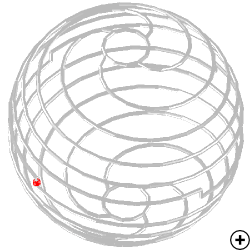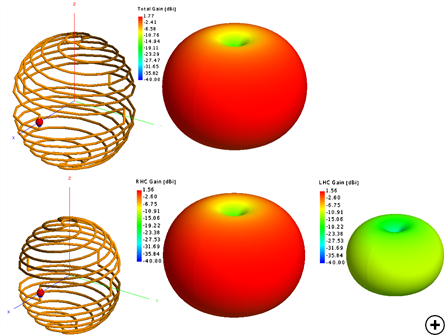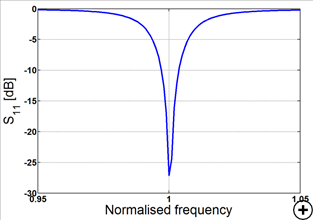Folded Spherical Helix DipoleImage of the Folded Spherical Helix Dipole.

The Folded Spherical Helix Dipole (FSHD) is an electrically small self-resonant antenna, which exhibits a good impedance match with a low Q at a value ka ~ 0.27, where k = 2*(pi/wavelength) and a is the radius of a sphere that can completely encapsulate the antenna. An antenna is considered electrically small if ka < 0.5.

For a 4-arm FSHD the input impedance is typically ~ 50 Ohms, but this can be adjusted by varying the number of helical arms and the number of turns. Furthermore the FSHD can be configured to either have linear or elliptical polarization by changing the direction of rotation of the helical arms between the two hemispheres.Typical radiation pattern at the centre frequency for a Linear (top) and RHC (bottom) polarised designTypical reflection coefficient versus frequency for a 4-arm FSHD